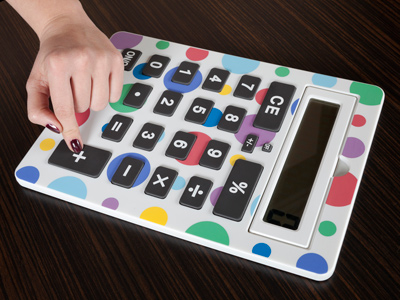# Addition and Subtraction 3 (Easy)

Welcome to the third of our Easy Eleven Plus maths quizzes on addition and subtraction. You may think that these questions are very simple, and you can do them in your head. You may be right, but it never hurts to practice your mental arithmetic skills! You’ll also find many hints and tips which will help you in your maths lessons, in real life situations, and in your 11+ exams.

One way to improve your addition and subtraction skills is by rounding numbers up or down. For example:

• To subtract 78, first subtract 80 then add 2

Can you see how that idea works? It’s a quick way to solve problems which may appear more complicated than they are.

Now on to the quiz. If you haven’t already played our previous ones on addition and subtraction, then go back and try them all until you always score a perfect ten-out-of-ten!

1.
74 - 98 + 29 = ?
201
-5
5
15
TIP: With numbers less than 100, add the tens first and then add the units.
74 - 98 + 29 = (70 + 20 - 90) + (4 + 9 - 8) = 0 + 5 = 5
A quick way to subtract 98 is to subtract 100 then add 2
2.
26 + 47 = ?
63
83
53
73
TIP: With numbers less than 100, add the tens first and then add the units.
26 + 47 = (20 + 40) + (6 + 7) = 60 + 13 = 73
Another way to add 47 is to add 50 then subtract 3: 26 + 50 = 76. 26 - 3 = 73
3.
54 - 27 = ?
26
27
28
29
TIP: With numbers less than 100, add the tens first and then add the units.
54 - 27 = (50 - 20) + (4 - 7) = 30 + -3 = 27
Another way to do this is to subtract 30 then add 3: 54 - 30 = 24. 24 + 3 = 27
4.
27 - 68 + 59 - 9 = ?
9
11
-9
7
TIP: With numbers less than 100, add the tens first and then add the units.
27 - 68 + 59 - 9 = (20 + 50 - 60) + (7 + 9 - 8 - 9) = 10 + -1 = 9
Remember: if you are adding a minus number, that’s the same as subtracting a positive number
5.
22 - 38 + 45 = ?
29
28
30
31
TIP: With numbers less than 100, add the tens first and then add the units.
22 - 38 + 45 = (20 + 40 - 30) + (2 + 5 - 8) = 30 + -1 = 29.
Remember: if you are adding a minus number, that’s the same as subtracting a positive number
6.
34 + 0 + 58 + 17 - 0 + 5 = ?
110
124
104
114
TIP: With numbers less than 100, add the tens first and then add the units.
34 + 0 + 58 + 17 - 0 + 5 = (30 + 50 + 10) + (4 + 8 + 7 + 5) = 90 + 24 = 114. Ignore zeros!
7.
47 + 15 + 73 = ?
125
135
145
155
TIP: With numbers less than 100, add the tens first and then add the units.
47 + 15 + 73 = (40 + 10 + 70) + (7 + 5 + 3) = 120 + 15 = 135
You may have noticed another shortcut: 7 + 3 = 10 so 47 + 73 = 40 + 70 + 10
8.
15 + 15 - 15 + 15 = ?
60
15
45
30
That was an example of a question you must read thoroughly - you didn't think all the 15s were to be added did you?
9.
23 + 37 + 53 = ?
103
113
93
123
TIP: With numbers less than 100, add the tens first and then add the units.
23 + 37 + 53 = (20 + 30 + 50) + (3 + 7 + 3) = 100 + 13 = 113
Don't forget: sometimes you will spot an easy addition which will make the calculation easier, e.g. 23 + 37 = 60
10.
6 - 12 + 27 + 33 = ?
58
56
54
52
TIP: With numbers less than 100, add the tens first and then add the units.
6 - 12 + 27 + 33 = (20 + 30 - 10) + (6 + 7 + 3 - 2) = 40 + 14 = 54
Be on the lookout for simple calculations that make your life easier! 27 + 33 is one of these
Author:  Frank Evans